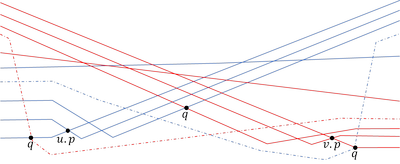# Maintaining the Union of Unit Discs Under Insertions with Near-Optimal Overhead

## AbstractFinding the intersection between two lower envelopes of pseudo-lines in O(log n) time, using tentative binary search. An example where it is unclear how to proceed with the search given existing information. The Solid Pseudo-lines are fixed. The Dashed pseudo-lines are optional, namely ,either none of the dashed pseudo-lines exists or exactly one of them exists. u.p andv.p are the current points during the search. The intersection q can be to the left of u.p, between u.p and v.p or to the right of v.p depending on the existing of the dashed lines.

We present efficient data structures for problems on unit discs and arcs of their boundary in the plane. (i) We give an output-sensitive algorithm for the dynamic maintenance of the union of n unit discs under insertions in O(k log2 n) update time and O(n) space, where k is the combinatorial complexity of the structural change in the union due to the insertion of the new disc. (ii) As part of the solution of (i) we devise a fully dynamic data structure for the maintenance of lower envelopes of pseudo-lines, which we believe is of independent interest. The structure has O(log2 n) update time and O(log n) vertical ray shooting query time. To achieve this performance, we devise a new algorithm for finding the intersection between two lower envelopes of pseudo-lines in O(log n) time, using tentative binary search; the lower envelopes are special in that at \em>x=-∞ any pseudo-line contributing to the first envelope lies below every pseudo-line contributing to the second envelope. (iii) We also present a dynamic range searching structure for a set of circular arcs of unit radius (not necessarily on the boundary of the union of the corresponding discs), where the ranges are unit discs, with O(n log n) preprocessing time, O(n1/2+ε + l) query time and O(log2 n) amortized update time, where l is the size of the output and for any ε>0. The structure requires O(n) storage space.